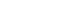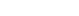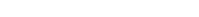This tutorial will show you how to get to any point on a line. It starts by introducing you to a line in two dimensions and showing you how this can be extended to take into account any point on a line in 3 dimensions.

## Common Methods: Given a point on the line and direction

1. Find the vector equation of the linepassing through the pointand parallel to the vector## Common Methods: Line passing through two given points

1. Find the vector equation of the line passing through the points